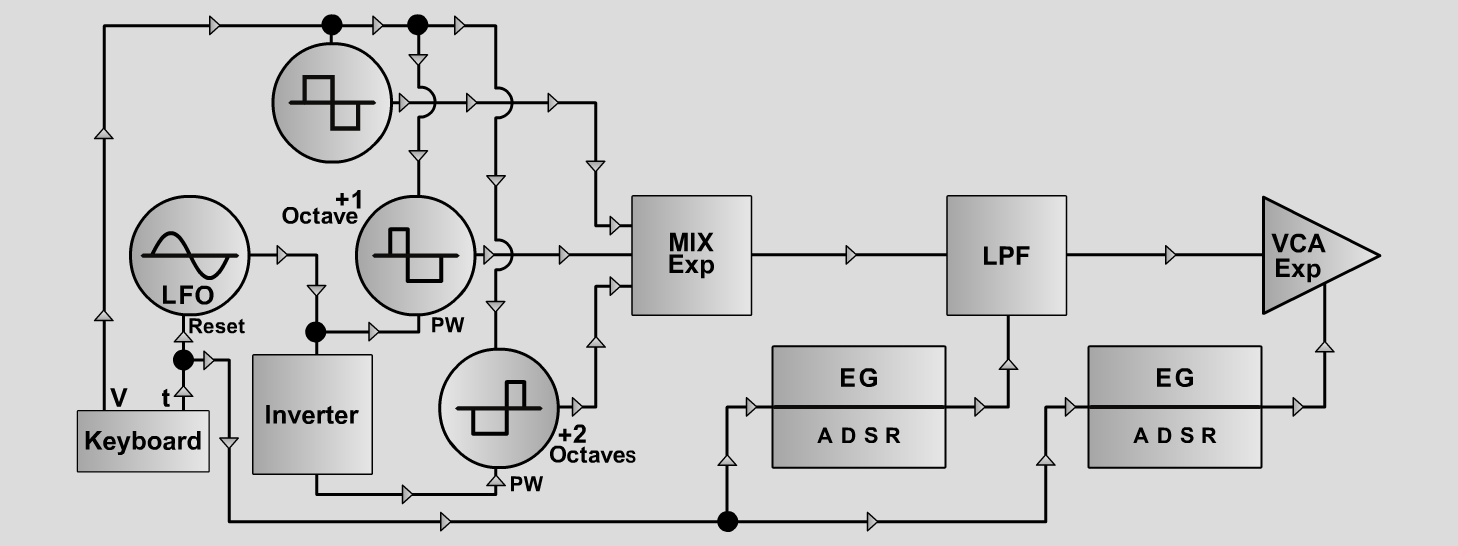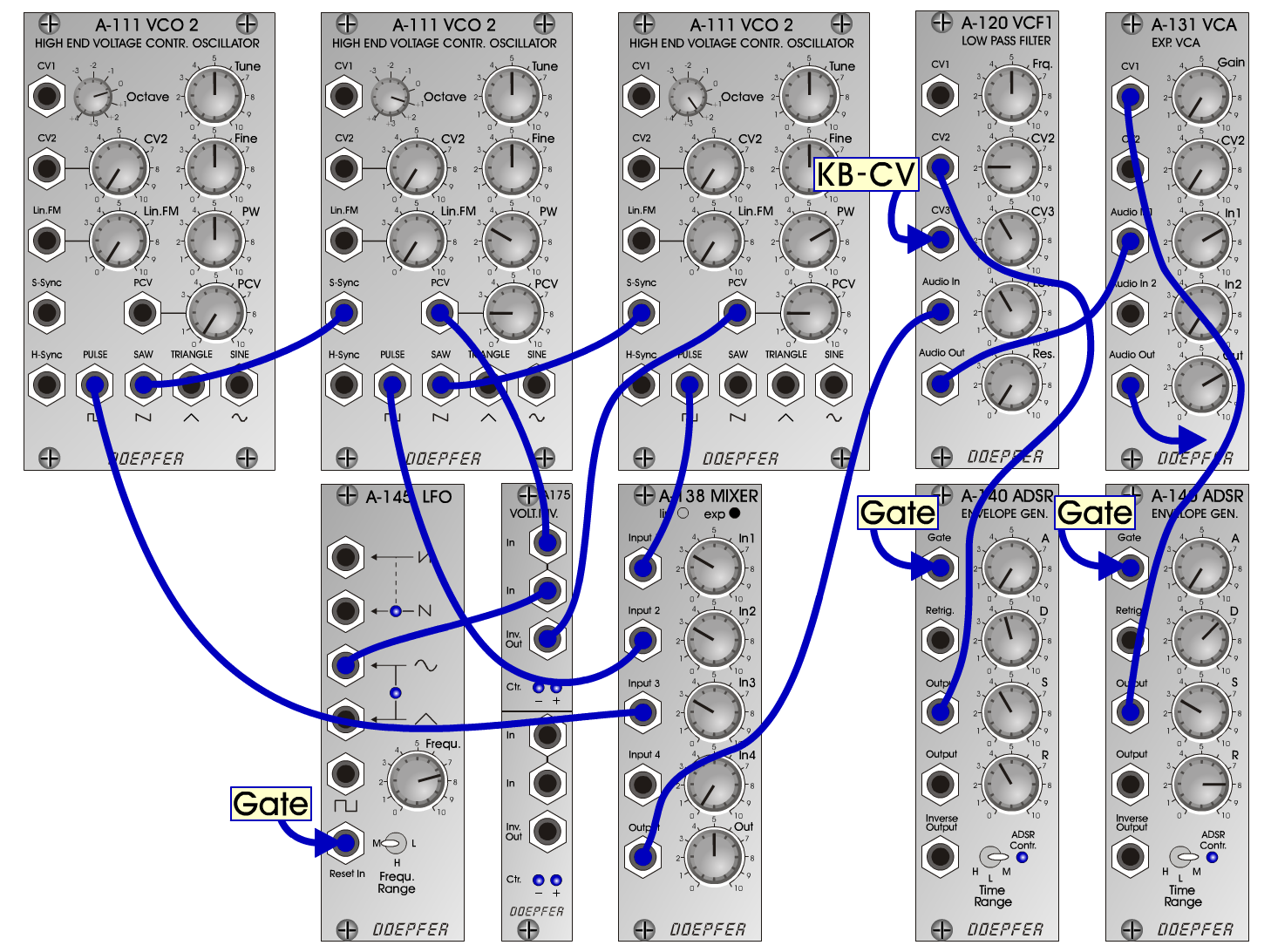ThreePulsesS y n t hD o e p f e r A - 100 Connections: Settings: ThreePulses 1 A-111/1 (Pulse) <=> A-138exp (Input 3)  A-111/2 (Pulse) <=> A-138exp (Input 2)  A-111/3 (Pulse) <=> A-138exp (Input 1)  A-138exp (Output) <=> A-120 (Audio In)  A-120 (Audio Out) <=> A-131 (Audio In)  A-131 (Audio Out) <=> Amp  A-145 (Sine) <=> A-175 (In1)  A-175 (In2) <=> A-111/2 (PCV)  A-175 (Inv. Out) <=> A-111/3 (PCV)  A-140/1 (Output) <=> A-120 (CV2)  Keyboard CV <=> A-120 (CV3)  A-140/2 (Output) <=> A-131 (CV1) A-111/1 (Octave = 0, PW = 5)  A-111/2 (Octave = 1, PW = 3, PCV = 2)  A-111/3 (Octave = 2, PW = 7, PCV = 2)  A-138 (In 1 = 3, In 2 = 3, In 3 = 3, Out = 5)  A-120 (Freq = 4.1, Lev = 10, Res = 0)  A-140/1 (A = 0.2, D = 0.2, S = 5, R = 10, Range = M)  A-140/2 (A = 0.2, D = 7.5, S = 7, R = 6, Range = M)  A-145 (Frequ. = 7.5, Range = M)  A-131 (Gain = 0, Audio In 1 = 6, Audio Out = 6) ThreePulses2 A-120 (Freq = 4, Lev = 10, Res = 0) ThreePulses3 A-120 (Freq = 6, Lev = 10, Res = 0) ThreePulses4 A-111/2 (Octave = 1, PW = 3, PCV = 4)  A-111/3 (Octave = 2, PW = 7, PCV = 4)  A-120 (Freq = 6, Lev = 10, Res = 0)  A-140/1 (A = 0.2, D = 4.5, S = 7, R = 4, Range = M)  A-140/2 (A = 0.2, D = 6.5, S = 7, R = 8, Range = M) Tuning: A-111/2 => + 1 octave; A-111/3 => + 2 octaves Josef MuellerSound samples Bydlo (excerpts) Bydlo (Mussorgsky: Pictures at an Exhibition)     (2. sound: PlasmaBass) ThreePulses 1 A ThreePulses 1 B ThreePulses 1 C ThreePulses 2 A ThreePulses 2 B ThreePulses 2 C ThreePulses 3 A ThreePulses 3 B ThreePulses 3 C ThreePulses 4 A ThreePulses 4 B ThreePulses 4 C